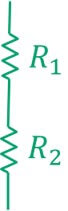# Basics of Electrical Engineering

Learn the basics of Electrical Engineering.

Series and Parallel arrangements are two basic configurations in which we can arrange the electrical components.
You'll learn:
• What is series circuit
• Basic properties of series circuits
• How to solve resistors in series circuits
• Examples to solve series circuits
• What is parallel configuration
• How to solve resistors in parallel circuits
• Basic properties of parallel circuits
• Examples to solve parallel resistor circuits
Let's take an overview of series vs parallel configuration:

### What is Series Configuration

A series circuit involves the simple arrangement of components in such way that one end of both components are joined together and there is no other connection in between them. The figure below displays two resistors R1 and Rin the series configuration.A series circuit always follows the above configuration. The figure below displays a series circuit with a voltage source and two resistors.#### Properties of Series Circuits

A series circuit has unique properties which make it distinct from the parallel. Let's understand them.

Current always remains same: The current in series circuit always remains same. If 5 A current is flowing through the R1 then the same current will flow through the R2.

Voltage divides in series circuits: The amount of voltage in series components is not same. Instead, the magnitude of voltage dropped across the individual components is dependent on the magnitude of their resistance. The voltage divider rule is used to calculate this drop.

Resistance is additive: The resistors in series are additive and one can replace multiple series resistors by a single equivalent resistor whose magnitude.

### How to solve resistors in series: With Examples

As explained above, the resistors are solved by simply adding them.
Req = R+ R+ R3... Rn
The figure below illustrates how this formula can be utilized for three series resistors of 5, 10, and 15 for obtaining their equivalent value.
Let's solve a more practical example where we are interested to find the current flowing through a series circuit. You'll also learn to apply the Ohm's law in series circuits.

Example # 1: Three resistors of 10 Ω, 20 Ω, and 30 Ω in series are powered by a 12 V battery. Find the current flowing through the circuit.
Solution:
Step 1: Find the Req = R+ R+ R3 = 10 Ω + 20 Ω + 30 Ω = 60 Ω
Step 2: Apply the Ohm's law to calculate current I = V/Req = 12 V / 60 Ω = 0.2 A

### What is parallel configuration

In the previous section, we observed the combination of components (resistors) which formed an electric circuit. The common point where two or more than two components meet is technically referred as the node. A dark spot or a dot is used to indicate the junction point (node) in circuits. The figure below displays a node formed between R1 and R2.
Two or more than two components are in parallel configuration when one end of all terminals share a common node, and the other ends of terminals share the other common node. The figure below displays another example of the parallel connection.
There is an alternate way to redraw the above schematic.
While the above two figures are essentially same the second figure doesn't contain a node. We can go both ways one can either indicate a node by a dot or just can connect the components in this configuration.

#### Properties of parallel circuits

Let's understand the behavior of current, voltage, and resistance in the parallel circuits.

Voltage always remains the same: The potential difference across all parallel components remains the same.

Current divides in parallel: The current entering any node divides among the parallel resistors. The magnitude of the divided current is always inversely related to the resistance. The current divider rule helps us to understand this behavior.

### How to solve parallel resistors

Two or more than two resistors can be solved in parallel by using the equation below:
Let's solve an example to understand this:

Example #1 Three parallel resistors of 6 Ω, 12 Ω, and 20 Ω are connected in parallel. Find the equivalent resistance.
Solution:
1/Req = 1/R1+ 1/R+ 1/R3. = [1/6 Ω] + [1/12 Ω] + [1/20 Ω] = 3.33 Ω

Let's solve another problem where we apply the Ohm's law to parallel circuits.

Example # 2: A parallel circuit contains two resistors whose resistance values are 20 Ω each. Find the overall current which is supplied by the 5 V source.
Solution:
Step 1: To find Req by 1/Req = 1/R1+ 1/R2 = [1/20 Ω] + [1/20 Ω] = 10 Ω
In the present case the Ohm's law will become:
I = V/R = 5 / 10 = 0.5 A

So that was all about Series vs Parallel Circuit configuration. Let's recap our discussion in tabulated form:
Property Series Circuits Parallel Circuits
How it looks

Current It always remains same in series. It divides in parallel.
Voltage It divides in series. Remains same in parallel.
Resistance Is additive: Req = R+ R+ R3... Rn Is solved by: [1/Req] =[1/ R1] + [1/R2] + [1/R3] ... [1/Rn]

#### 7 comments:

1.I really appreciate your explanation, but I am wondering if there is a mistake in the the parallel circuit example #2 where the resistance is calculated for resistors with of 20 Ohm each. Should R(eq) not be 10 Ohm, instead of 3.33? It it maybe a copy and paste error from the previous example?

1.3.3 is correct (60/18 )

2.Yeah that is 10 ohm. Corrected the mistake.

3.in the table given above, correction required for Voltage divides in series and remains same in parallel

4.Table is incorrect

5.this is for the parallel circuit of 6 ohms, 12 ohms and 20 ohms resistors

6.Printed circuit boards (PCBs) are the boards that are used as the base in most electronics – both as a physical support piece and as the wiring area for the surface-mounted and socketed components.  https://www.pcbonline.com/